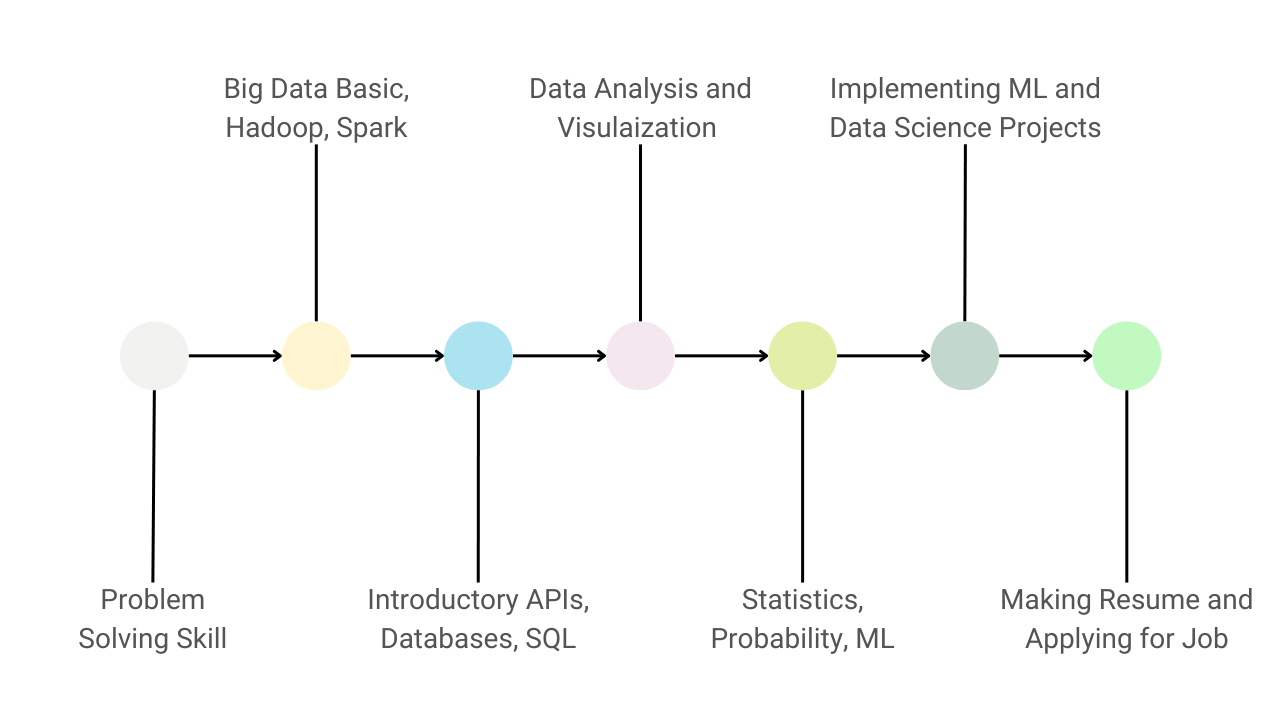# machine-learning-guidanceGuide to Learn Data Science and Become a Data Scientist

Data Science is a sought-after career option attracting many fresh graduates. The path to becoming a successful data scientist involves following a structured sequence of steps. We have outlined the journey in 7 concise steps and presented a data science job description to make the discussion realistic.Introduction to Scikit-Learn

Scikit-learn is a free machine learning framework available for Python, providing an interface for supervised and unsupervised learning. It is built over the SciPy library and provides every feature catering to every ML requirement. In this blog, we will learn the essential concepts, tools, and features related to Scikit-learn.Step by Step Guide to Get Started with Machine Learning

In this blog, we have discussed steps to master machine learning for implementing applications. Here are the steps: 1) Learn python 2) Learn math for ML 3) Learn concept of data, analysis, manipulation, filtering, and visualization and choose any open source dataset 5) Learn basics of ML and choose problem statement 6) Learn deep learning and neural networks.Introduction to Machine Learning

Machine learning is the science of getting computers to act without being explicitly programmed. Here computer takes Input Data and Output Data as its input parameters and tries to produce the best suitable function that maps Inputs to Outputs. The machine learns a mapping function that maps the input data to the output using existing experiences.There are various ways to make our computers machine learning enabled for implementing ML projects. In this blog, we will try one of the most preferred and easy-to-use methods, i.e., Python3 with Sublime Text 3. Python programming is the most preferred language for ML tasks, and sublime text 3 is the code editor to write ML codes.Top Challenges Faced by Machine Learning Beginners

Many learners want to master machine learning and don’t know where to start. It seems like a formidable task, especially if one lacks a thorough background. This article will discuss some of the factors that can be obstacles in learning machine learning. Working around these obstacles can help us to develop a long-term interest in this subject.Top Misconceptions in Machine Learning

We sometimes overemphasize the capabilities of machine learning. Here are some popular misconceptions: 1) ML, DL, and AI are the same 2) Machine learning can predict the future 3) Machine learning can solve any problem 4) One machine learning algorithm will be sufficient 5) More features in the data, better will be the machine learning model, etc.Top Historical Facts in ML

To learn a new subject, we should try to know how exactly that started. Every computer science field has a different history, reflecting the challenges that earlier researchers faced and making our journey easy. This article will discuss the 10 most interesting historical facts considered the turning points in AI and Machine Learning history.Why DSA Important for Machine Learning?

In this blog, we have explained the top five reasons to learn data structures and algorithms for data science and machine learning. Some popular DSA concepts used in machine learning are array, vector, matrices, linked list, tree, graph, stack, queue, hashing, sets, dynamic programming, greedy algorithm, randomized algorithms, etc.How Machine Learns in Machine Learning?

In Machine Learning, a machine learns by using algorithms and statistical models to identify patterns in data. Here the process of learning begins with feeding a large amount of training data to the algorithm. The algorithm then uses this data to make predictions or take actions based on the patterns it has identified. In other words, the algorithm constantly adjusts its parameters to minimize the difference between its predictions and actual outcomes.Machine Learning Glossary (A to Z Terms) in ML

This is a glossary of machine learning terms commonly used in the industry. Some popular machine learning terminologies: neural networks, supervised learning, unsupervised learning, reinforcement learning, regularization, classification, regression, clustering, optimizers, outliers, bias, variance, underfitting, overfitting, normalization, etc.Introduction to Machine Learning

Machine learning is the science of getting computers to act without being explicitly programmed. In this blog, we have answered these fundamental questions: 1) What is machine learning and how it works? 2) Why do we need machine learning? 3) When did it start? 4) Use cases of machine learning in industry 5) Machine learning vs. Artificial intelligence